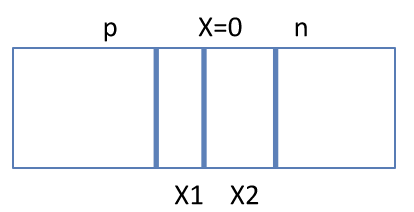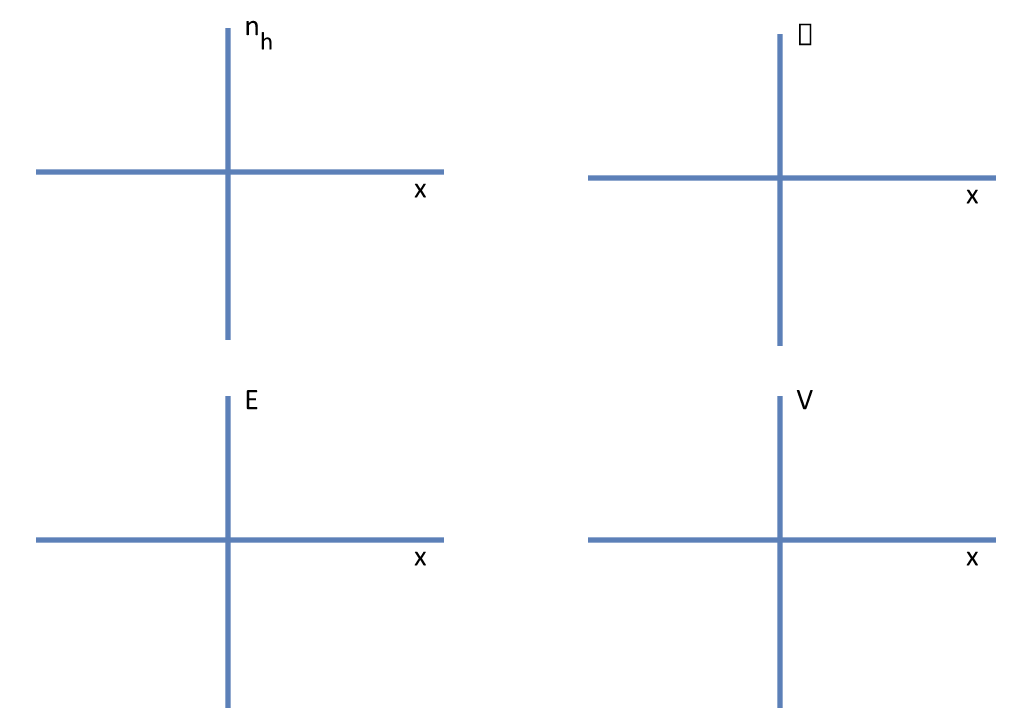# Thought provoking problems on modern physics

These problems are from pre-test conducted in teacher's workshops by Prof HC Verma and his team.

## Test 1

Source: RESPECT KV MRP Workshop 15th-24th May 2017
1. The electric field in a metal is zero in electrostatics. But in Rutherford's experiment, the alpha particle gets deflected showing presence of electric field there. This is because,
1. Gold is not a metal but semi metal
2. When alpha particle moves in gold, this is not an electrostatic situation.
3. The moving alpha particle creates a magnetic field which shifts the electron clouds to create the electric field.
4. The electric field inside a metal is zero only in an average sense.
5. The alpha particles are deflected from the surface of the gold foil and at the surface of a metal.
2. A helium nucleus is stable because the nuclear attractive force dominates over Coulomb repulsion. Why 4 neutrons do not make a stable nucleus where there should be a strong nuclear attractive force but no Coulomb repulsion.
3. A gamma decay is most often preceded by either alpha decay or beta decay. This because
1. gamma rays are produced by alpha or beta particles
2. a gamma decay alone does not conserve momentum conservation
3. in alpha or beta decay, the daughter nucleus is prepared in excited state
4. in nuclear reactions, the energy released is so large that gamma ray alone cannot take up this energy alone.
4. A voltage is applied to a hydrogen discharge tube just enough to produce a faint orange light. Together with this,
1. a lots of ultraviolet radiation must be coming
2. a lots of infrared radiation must be coming
3. a lots of visible light of different wavelengths must be coming.
5. In Bohr's model, the angular momentum of the electron in hydrogen atom for $n=1$ orbit is Planck's constant/($2\pi$). But this electron is also called 1s electron having angular momentum $l = 0$. Which of the two is more realistic?
6. A photo cell is exposed to light of frequency n1 in one experiment and of a higher frequency n2 in another experiment. The intensity of light is kept same in the two cases.
1. In which case, the number of photons falling on the cathode is more? Or it is same in the two cases?
2. Sketch the photocurrent I vs anode voltage for the two cases.
7. A pn junction is schematically shown in the figure. The depletion region extends for a width x1 on the p-side and for a width x2 on the n-side.
8.1. x1 must be equal to x2.
2. p-type extends from the left end to x = -x1 and n-type extends from x=x2 to the right end.
3. Sketch the graph of the following as a function of x. (i) $n_h$ (number of holes per unit volume), (ii) charge density $\rho$, (iii) electric field $E$, and (iv) potential (taking V = 0 at x = 0).